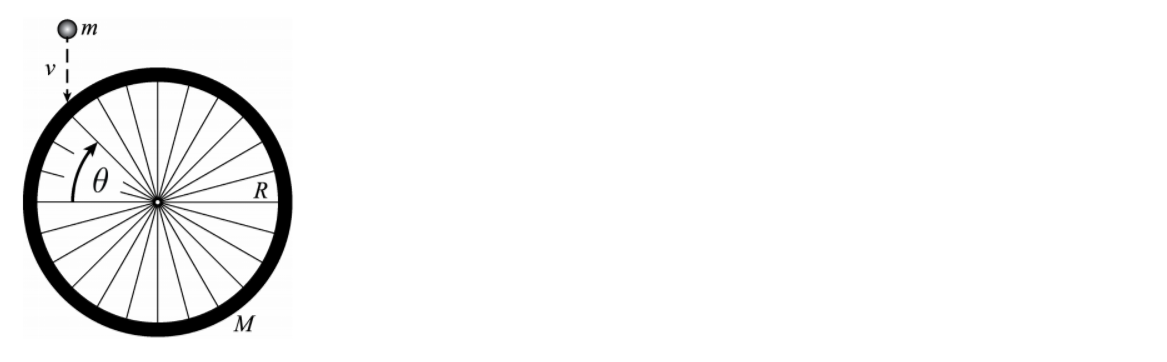Problem: A stationary bicycle wheel of radius R is mounted in the vertical plane on a horizontal low friction axle. Initially the wheel is not rotating. The wheel has mass M, all concentrated in the rim (spokes have negligible mass). A lump of clay with mass m falls and sticks to the outer edge of the wheel at an angle θ as shown in the figure. Just before impact the clay has a speed v. Just after impact, what is the magnitude of the angular velocity of the wheel?1. mvcosθ / MR2. mv / (m + M)R3. mv / MR4. mvcosθ / (m + M)R5. mvsinθ / (m + M)R6. mv / (m + M)R 27. Mv / mR8. Mvsinθ / mR9. mv / MR 210. mvsinθ / MR

96% (69 ratings)
Problem Details

A stationary bicycle wheel of radius R is mounted in the vertical plane on a horizontal low friction axle. Initially the wheel is not rotating. The wheel has mass M, all concentrated in the rim (spokes have negligible mass). A lump of clay with mass m falls and sticks to the outer edge of the wheel at an angle θ as shown in the figure. Just before impact the clay has a speed v. Just after impact, what is the magnitude of the angular velocity of the wheel?

1. mvcosθ / MR

2. mv / (m + M)R

3. mv / MR

4. mvcosθ / (m + M)R

5. mvsinθ / (m + M)R

6. mv / (m + M)R 2

7. Mv / mR

8. Mvsinθ / mR

9. mv / MR 2

10. mvsinθ / MR